# Suppose X and Y are independent Binomial random variables, each with n=3 and p=9/10. a. Find...

Suppose X and Y are independent Binomial random variables, each with n=3 and p=9/10.

a. Find the probability that X and Y are equal, i.e., find P(X=Y).

b. Find the probability that X is strictly larger than Y, i.e., find P(X>Y).

c. Find the probability that Y is strictly larger than X, i.e., find P(Y>X).

a) The probability here is computed as: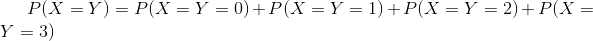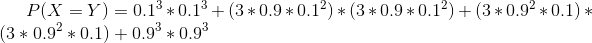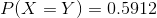Therefore 0.5912 is the required probability here.

b) Now there are two possibilities here, either X > Y or Y > X in case where X is not equal to Y.

P(X not equal to Y) = 1 - P(X = Y) = 1 - 0.5912 = 0.4088

Therefore due to symmetry we would have: P(X > Y) = P(Y > X) = 0.4088 / 2 = 0.2044

Therefore 0.2044 is the required probability here.

c) As explained in the previous part itself, P(X > Y) = P(Y > X) = 0.2044

Therefore 0.2044 is the required probability here.

#### Earn Coin

Coins can be redeemed for fabulous gifts.

Similar Homework Help Questions
• ### Let X, Y be independent random variables where X is binomial(n = 4, p = 1/3)...

Let X, Y be independent random variables where X is binomial(n = 4, p = 1/3) and Y is binomial(n = 3,p = 1/3). Find the moment-generating functions of the three random variables X, Y and X + Y . (You may look up the first two. The third follows from the first two and the behavior of moment-generating functions.) Now use the moment-generating function of X + Y to find the distribution of X + Y .

• ### Suppose that we have two independent binomial random variables X ~Binomial(n, px) and Y ~ Binomial(m,Pv)....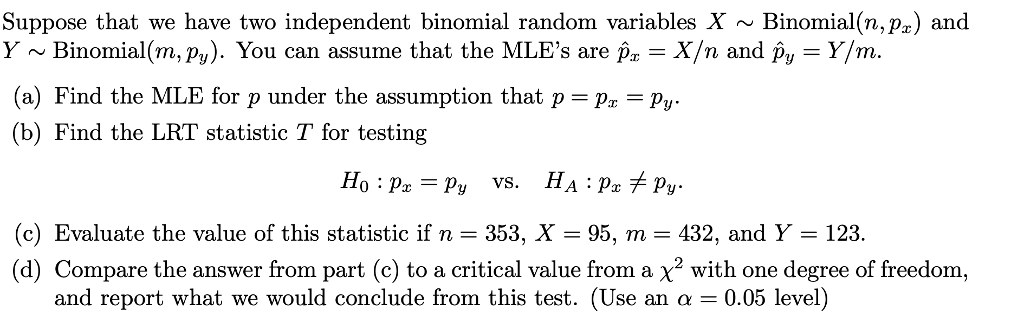Suppose that we have two independent binomial random variables X ~Binomial(n, px) and Y ~ Binomial(m,Pv). You can assume that the MLE's are -X/n and p,-Y/m. (a) Find the MLE for p under the assumption that p (b) Find the LRT statistic T for testing p,-py HA:p.Ру vs. (c) Evaluate the value of this statistic if n 353, X 95, m -432, and Y 123. (d) Compare the answer from part (c) to a critical value from a x2 with...

• ### Let X and Y be independent binomial random variables B(n,p) on the same sample space. Show that X + Y is also a binomial...

Let X and Y be independent binomial random variables B(n,p) on the same sample space. Show that X + Y is also a binomial random variable B(?,?). What values should replace the questions marks?

• ### Let x be the binomial random variable with n=10 and p = 9 a. Find P(x...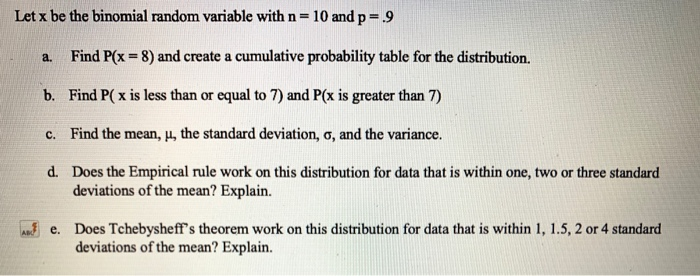Let x be the binomial random variable with n=10 and p = 9 a. Find P(x = 8) and create a cumulative probability table for the distribution. b. Find P( x is less than or equal to 7) and P(x is greater than 7) c. Find the mean, u, the standard deviation, o, and the variance. d. Does the Empirical rule work on this distribution for data that is within one, two or three standard deviations of the mean? Explain....

• ### Could you help me solve this question 9. X and Y are two independent random variables...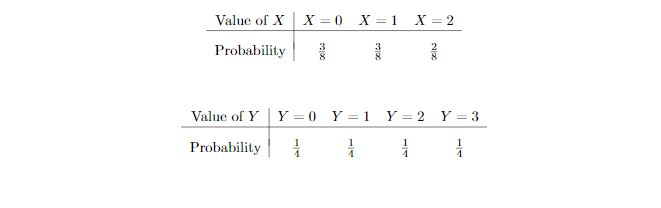Could you help me solve this question 9. X and Y are two independent random variables defined as in the "Graphs Document". What is P(X <= Y)? (i.e., the probability that X is not larger than Y. Hint: Draw the probability tree.) = x=0 X=1 Value of x Probability X = 2 : Value of Y Probability Y = 0 Y=1 Y = 2 Y = 3 i i i

• ### 1. Suppose that random variables X and Y are independent and have the following properties: E(X)...

1. Suppose that random variables X and Y are independent and have the following properties: E(X) = 5, Var(X) = 2, E(Y ) = −2, E(Y 2) = 7. Compute the following. (a) E(X + Y ). (b) Var(2X − 3Y ) (c) E(X2 + 5) (d) The standard deviation of Y . 2. Consider the following data set: �x = {90, 88, 93, 87, 85, 95, 92} (a) Compute x¯. (b) Compute the standard deviation of this set. 3....

• ### 3. Suppose Xi, X2, and X are independent random variables drawn from a binomial distribution with parameters p and n. The observed values are Xi -3, X2-4, and (a) Suppose n 12 and p is unknown. What...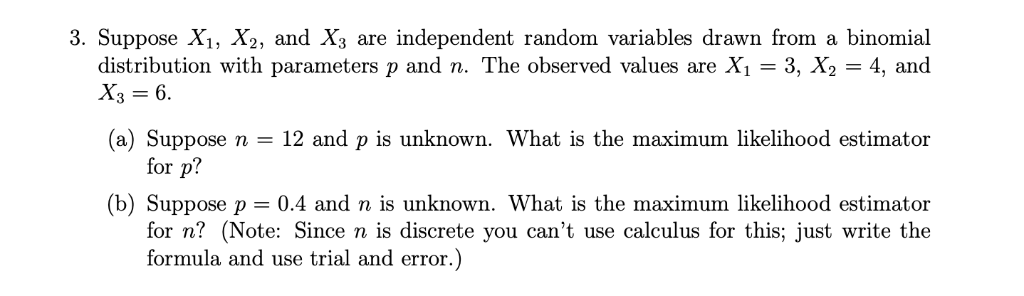3. Suppose Xi, X2, and X are independent random variables drawn from a binomial distribution with parameters p and n. The observed values are Xi -3, X2-4, and (a) Suppose n 12 and p is unknown. What is the maximum likelihood estimator (b) Suppose p - 0.4 and n is unknown. What is the maximum likelihood estimator for p? for n? (Note: Since n is discrete you can't use calculus for this; just write the formula and use trial and...

• ### 3. Suppose that we have two independent binomial random variablesXBinomial(n,pr) and Y~Binomial(m, p). You can assume...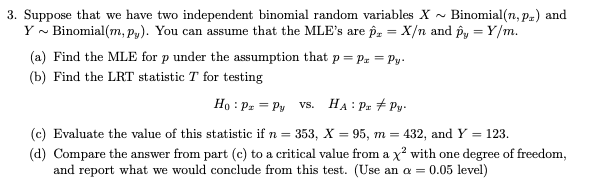3. Suppose that we have two independent binomial random variablesXBinomial(n,pr) and Y~Binomial(m, p). You can assume that the MLE's are p X/n and py- Y/m. (a) Find the MLE for p under the assumption that pr -Py (b) Find the LRT statistic T for testing (c) Evaluate the value of this statistic if n 353, X 95, m 432, and Y 123. (d) Compare the answer from part (c) to a critical value from a x2 with one degree of...

• ### Problem 41.3 Let X and Y be independent random variables each geometrically distributed with parameter p,...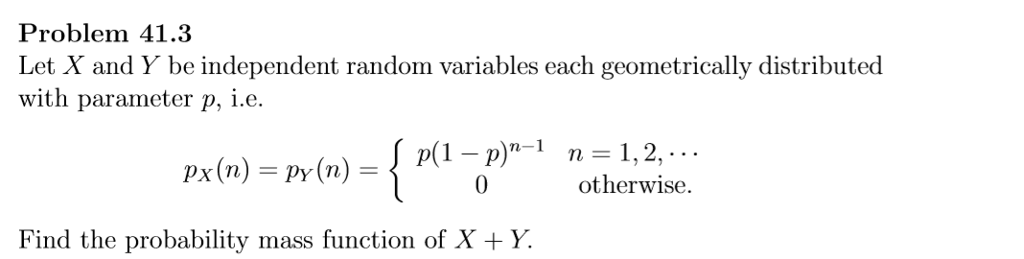Problem 41.3 Let X and Y be independent random variables each geometrically distributed with parameter p, i.e. p(1- p otherwise. Find the probability mass function of X +Y

• ### 2. Suppose X and Y are independent continuous random variables. Show that P(Y < X) =...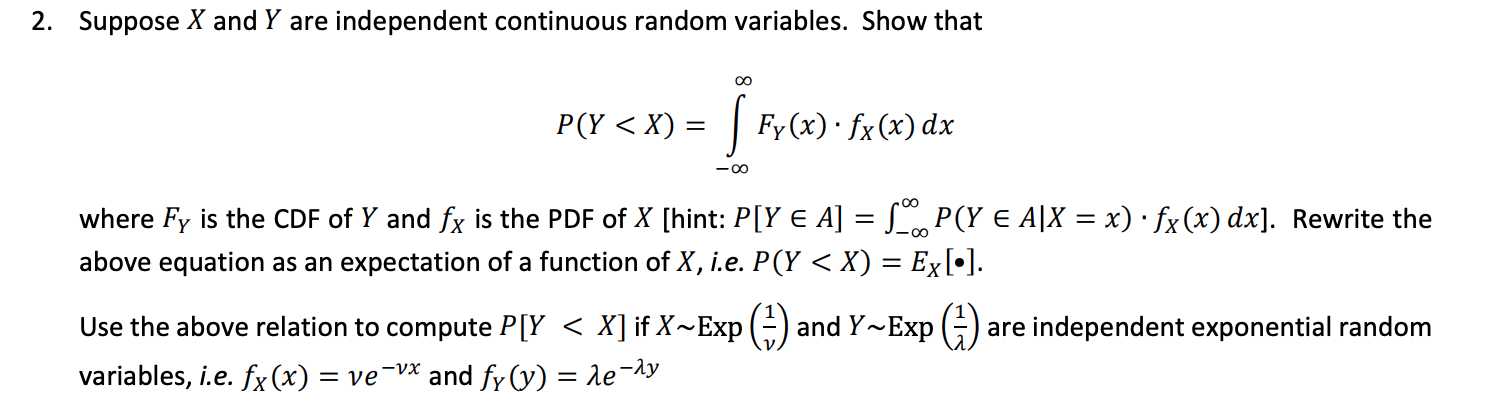2. Suppose X and Y are independent continuous random variables. Show that P(Y < X) = | Fy(x) · fx (x) dx -oo where Fy is the CDF of Y and fx is the PDF of X [hint: P[Y E A] = S.P(Y E A|X = x) · fx(x) dx]. Rewrite the above equation as an expectation of a function of X, i.e. P(Y < X) = Ex[•]. Use the above relation to compute P[Y < X] if X~Exp (2)...